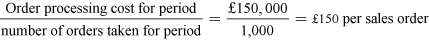### Become an OU studentStart this free course now. Just create an account and sign in. Enrol and complete the course for a free statement of participation or digital badge if available.

# 2.2.1 Tracing of costs to customers

In order to apply the activity-based costing approach it is necessary to identify the cost driver for a particular indirect cost and then establish a cost driver rate for charging the cost to a particular ‘cost object’, be it a product or customer. For example, the cost driver for sales order processing cost might be the number of orders taken. If the total sales order processing cost for a period is £150,000 and the number of orders taken is 1,000, then the cost driver rate will be:Equation 1

Order processing costs should then be allocated to customers at a rate of £150 for every sales order received from the customer.

## Activity 4 Calculate customer profitability using activity based costing

Timing: Spend about 25 minutes on this activity.

Alpha Ltd has three customers: Beta, Gamma and Delta. The management accountant has collected the following data concerning sales, transactions and costs relating to the three customers, as follows:

Table 3
Sales revenue£120,000£150,000£90,000
Number of orders placed136639
Number of units sold300500450
Warehouse floor space occupied (square metres) 30 40 25
Table 4
Alpha’s indirect costs and cost drivers
£
Order taking (number of orders taken)1,534.00
Packing (number of units packed)5,250.00
Dispatch (number of units dispatched)4,312.50
Warehousing (cost of floor space occupied by goods)1,007.00
Account administration (number of orders taken)2,242.00

The gross profit on sales (based on the standard profit margin) is 30%. The directly traceable service and support costs (consisting of selling, delivery, financing and settlement discounts) for each customer are as follows.

Table 5
Beta:£13,120
Gamma:£18,476
Delta:£13,620

Using activity based costing, calculate the net profit for each of the three customers.

Note: You can assume that the number of units packed is the same as the number of units dispatched/sold.

Table 6 Calculation of cost driver rates
Order taking = £1,534/118= £13.00 per order
Packing = £5,250/1,250= £4.20 per unit
Dispatch = £4,312.50/1,250= £3.45 per unit
Warehousing = £1,007/95= £10.60 per square metre of floor space
Account administration = £2,242/118= £19.00 per order
Table 7 Customer activity costs
£££

Ord. taking

13 × £13 =16966 × £13 =85839 × £13 =507

Packing

300 × £4.20 =1,260500 × £4.20 =2,100450 × £4.20 =1,890

Dispatch

300 × £3.45 =1,035500 × £3.45 =1,725450 × £3.45 =1,553

W’housing

30 × £10.60 =31840 × £10.60 =42425 × £10.60 =265

13 × £19.00 =Total 24766 × £19.00 =Total 1,25439 × £19.00 =Total 741

Total act’y

traced costs

Total 3,029Total 6,361Total 4,956
Table 8 Customer profitability analysis
£££
Sales revenue120,000150,00090,000
Gross profit (30% of sales)36,00045,00027,000
Directly traceable service/support costs13,12018,47613,620
Total activity costs (as per above analysis)3,0296,3614,956
Net profitTotal 19,851Total 20,163Total 8,424

### Discussion

The calculations show that the ranking of customers in terms of total profit is the same as in terms of sales revenue:

Table 9
Revenue120,000150,00090,000
Ranking213
Net profit19,85120,1638,424
Ranking213

However, if profitability in terms of profit as a percentage of sales revenue is considered, a different picture emerges:

Table 10
Revenue120,000150,00090,000
Ranking213
Net profit as % of revenue16.5%13.4%9.4%
Ranking123

This is because both indirect costs and directly traceable service and support costs are (proportionately) highest for Delta and lowest for Beta (with Gamma in between):

Table 11